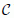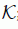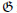# On properties of k-quasi-class $A\left(n\right)$ operators

## Abstract

Let n and k be positive integers; an operator $T\in B\left(\mathcal{H}\right)$ is called a k-quasi-class $A\left(n\right)$ operator if ${T}^{\ast k}\left({|{T}^{1+n}|}^{\frac{2}{1+n}}-{|T|}^{2}\right){T}^{k}\ge 0$, which is a common generalization of class A and class $A\left(n\right)$ operators. In this paper, firstly we prove some basic structural properties of this class of operators, showing that if T is a k-quasi-class $A\left(n\right)$ operator, then the nonzero points of its point spectrum and joint point spectrum are identical, the eigen-spaces corresponding to distinct eigenvalues of T are mutually orthogonal, the nonzero points of its approximate point spectrum and joint approximate point spectrum are identical; secondly we consider the tensor products for k-quasi-class $A\left(n\right)$ operators, giving a necessary and sufficient condition for $T\otimes S$ to be a k-quasi-class $A\left(n\right)$ operator when T and S are both nonzero operators.

MSC:47B20, 47A63.

## 1 Introduction

Let be a separable complex Hilbert space andbe the set of complex numbers. Let $B\left(\mathcal{H}\right)$ denote the ${C}^{\ast }$-algebra of all bounded linear operators acting on . Recall that $T\in B\left(\mathcal{H}\right)$ is called p-hyponormal for $p>0$ if ${\left({T}^{\ast }T\right)}^{p}-{\left(T{T}^{\ast }\right)}^{p}\ge 0$ ; when $p=1$, T is called hyponormal. T is called paranormal if ${\parallel Tx\parallel }^{2}\le \parallel {T}^{2}x\parallel \parallel x\parallel$ for all $x\in \mathcal{H}$ [2, 3]. T is called normaloid if $\parallel {T}^{n}\parallel ={\parallel T\parallel }^{n}$ for all $n\in \mathbb{N}$ (equivalently, $\parallel T\parallel =r\left(T\right)$, the spectral radius of T). In order to discuss the relations between paranormal and p-hyponormal and log-hyponormal operators (T is invertible and $log{T}^{\ast }T\ge logT{T}^{\ast }$), Furuta et al.  introduced a very interesting class of operators: class A defined by $|{T}^{2}|-{|T|}^{2}\ge 0$, where $|T|={\left({T}^{\ast }T\right)}^{\frac{1}{2}}$, which is called the absolute value of T and they showed that class A is a subclass of paranormal and contains p-hyponormal and log-hyponormal operators. Recently Yuan and Gao  introduced class $A\left(n\right)$ (i.e., ${|{T}^{1+n}|}^{\frac{2}{1+n}}\ge {|T|}^{2}$) operators and n-paranormal operators (i.e., ${\parallel {T}^{1+n}x\parallel }^{\frac{1}{1+n}}\ge \parallel Tx\parallel$ for every unit vector $x\in \mathcal{H}$) for some positive integer n. For more interesting properties on class $A\left(n\right)$ and n-paranormal operators, see .

Let ,be complex Hilbert spaces and $\mathcal{H}\otimes \mathcal{K}$ the tensor product of ,; i.e., the completion of the algebraic tensor product of ,with the inner product $〈{x}_{1}\otimes {y}_{1},{x}_{2}\otimes {y}_{2}〉=〈{x}_{1},{x}_{2}〉〈{y}_{1},{y}_{2}〉$ for ${x}_{1},{x}_{2}\in \mathcal{H}$, ${y}_{1},{y}_{2}\in \mathcal{K}$. Let $T\in B\left(\mathcal{H}\right)$ and $S\in B\left(\mathcal{K}\right)$. $T\otimes S\in B\left(\mathcal{H}\otimes \mathcal{K}\right)$ denotes the tensor product of T and S; i.e., $\left(T\otimes S\right)\left(x\otimes y\right)=Tx\otimes Sy$ for $x\in \mathcal{H}$, $y\in \mathcal{K}$.

Definition 1.1 $T\in B\left(\mathcal{H}\right)$ is called a k-quasi-class $A\left(n\right)$ operator for positive integers n and k if

${T}^{\ast k}\left(|{T}^{1+n}{|}^{\frac{2}{1+n}}-{|T|}^{2}\right){T}^{k}\ge 0.$

In general, the following implications hold:

In this paper, firstly we prove some basic structural properties of this class of operators, showing that if T is a k-quasi-class $A\left(n\right)$ operator, then the nonzero points of its point spectrum and joint point spectrum are identical, the eigen-spaces corresponding to distinct eigenvalues of T are mutually orthogonal, the nonzero points of its approximate point spectrum and joint approximate point spectrum are identical; secondly we consider the tensor products for k-quasi-class $A\left(n\right)$ operators, giving a necessary and sufficient condition for $T\otimes S$ to be a k-quasi-class $A\left(n\right)$ operator when T and S are both nonzero operators.

## 2 The basic properties for k-quasi-class $A\left(n\right)$ operators

In the following lemma, we study the matrix representation of a k-quasi-class $A\left(n\right)$ operator with respect to the direct sum of $\overline{ran\left({T}^{k}\right)}$ and its orthogonal complement.

Lemma 2.1 Let $T\in B\left(\mathcal{H}\right)$ be a k-quasi-class $A\left(n\right)$ operator for positive integers n and k, and let $T=\left(\begin{array}{cc}{T}_{1}& {T}_{2}\\ 0& {T}_{3}\end{array}\right)$ on $\mathcal{H}=\overline{ran\left({T}^{k}\right)}\oplus ker{T}^{\ast k}$ be $2×2$ matrix expression. Assume that $ran{T}^{k}$ is not dense, then ${T}_{1}$ is a class $A\left(n\right)$ operator on $\overline{ran\left({T}^{k}\right)}$ and ${T}_{3}^{k}=0$. Furthermore, $\sigma \left(T\right)=\sigma \left({T}_{1}\right)\cup \left\{0\right\}$.

Proof Consider the matrix representation of T with respect to the decomposition $\mathcal{H}=\overline{ran\left({T}^{k}\right)}\oplus ker{T}^{\ast k}$: $T=\left(\begin{array}{cc}{T}_{1}& {T}_{2}\\ 0& {T}_{3}\end{array}\right)$. Let P be the orthogonal projection of onto $\overline{ran\left({T}^{k}\right)}$. Then ${T}_{1}=TP=PTP$. Since T is a k-quasi-class $A\left(n\right)$ operator, we have

$P\left(|{T}^{1+n}{|}^{\frac{2}{1+n}}-{|T|}^{2}\right)P\ge 0.$

Then

$|{T}_{1}^{1+n}{|}^{\frac{2}{1+n}}={\left({\left(TP\right)}^{\ast \left(1+n\right)}{\left(TP\right)}^{\left(1+n\right)}\right)}^{\frac{1}{1+n}}={\left(P|{T}^{1+n}{|}^{2}P\right)}^{\frac{1}{1+n}}\ge P|{T}^{1+n}{|}^{\frac{2}{1+n}}P$

by Hansen’s inequality . On the other hand

$|{T}_{1}{|}^{2}={T}_{1}^{\ast }{T}_{1}=P{T}^{\ast }TP=P{|T|}^{2}P\le P|{T}^{1+n}{|}^{\frac{2}{1+n}}P.$

Hence

$|{T}_{1}^{1+n}{|}^{\frac{2}{1+n}}\ge {|{T}_{1}|}^{2}.$

That is, ${T}_{1}$ is a class $A\left(n\right)$ operator on $\overline{ran\left({T}^{k}\right)}$.

For any $x=\left({x}_{1},{x}_{2}\right)\in \mathcal{H}$,

$〈{T}_{3}^{k}{x}_{2},{x}_{2}〉=〈{T}^{k}\left(I-P\right)x,\left(I-P\right)x〉=〈\left(I-P\right)x,{T}^{\ast k}\left(I-P\right)x〉=0,$

which implies ${T}_{3}^{k}=0$.

Since $\sigma \left(T\right)\cup \mathfrak{G}=\sigma \left({T}_{1}\right)\cup \sigma \left({T}_{3}\right)$, whereis the union of the holes in $\sigma \left(T\right)$, which happen to be a subset of $\sigma \left({T}_{1}\right)\cap \sigma \left({T}_{3}\right)$ by [, Corollary 7], $\sigma \left({T}_{3}\right)=0$, and $\sigma \left({T}_{1}\right)\cap \sigma \left({T}_{3}\right)$ has no interior points, we have $\sigma \left(T\right)=\sigma \left({T}_{1}\right)\cup \left\{0\right\}$.

In , Yuan and Ji introduced $\left(n,k\right)$-quasiparanormal operators. $T\in B\left(\mathcal{H}\right)$ is called a $\left(n,k\right)$-quasiparanormal operator for positive integers n and k if

${\parallel {T}^{1+n}\left({T}^{k}x\right)\parallel }^{\frac{1}{1+n}}{\parallel {T}^{k}x\parallel }^{\frac{n}{1+n}}\ge \parallel T\left({T}^{k}x\right)\parallel$

for $x\in \mathcal{H}$. □

In the following we give the relations between $\left(n,k\right)$-quasiparanormal and k-quasi-class $A\left(n\right)$ operators.

Theorem 2.2 Let T be a k-quasi-class $A\left(n\right)$ operator for positive integers n and k. Then T is a $\left(n,k\right)$-quasiparanormal operator.

To give a proof of Theorem 2.2, the following famous inequality is needed.

Lemma 2.3 (Hölder-McCarthy’s inequality )

Let $A\ge 0$. Then the following assertions hold:

1. (1)

$〈{A}^{r}x,x〉\ge {〈Ax,x〉}^{r}{\parallel x\parallel }^{2\left(1-r\right)}$ for $r>1$ and all $x\in \mathcal{H}$.

2. (2)

$〈{A}^{r}x,x〉\le {〈Ax,x〉}^{r}{\parallel x\parallel }^{2\left(1-r\right)}$ for $r\in \left[0,1\right]$ and all $x\in \mathcal{H}$.

Proof of Theorem 2.2 Suppose that T is k-quasi-class $A\left(n\right)$ operator. Then

${T}^{\ast k}\left(|{T}^{1+n}{|}^{\frac{2}{1+n}}-{|T|}^{2}\right){T}^{k}\ge 0.$

Let $x\in \mathcal{H}$. Then by Hölder-McCarthy’s inequality, we have

$\begin{array}{rcl}{\parallel {T}^{k+1}x\parallel }^{2}& =& 〈{T}^{\ast k}{|T|}^{2}{T}^{k}x,x〉\\ \le & 〈{T}^{\ast k}|{T}^{1+n}{|}^{\frac{2}{1+n}}{T}^{k}x,x〉\\ =& 〈{\left({T}^{\ast \left(1+n\right)}{T}^{1+n}\right)}^{\frac{1}{1+n}}{T}^{k}x,{T}^{k}x〉\\ \le & {〈{T}^{\ast \left(1+n\right)}{T}^{1+n}{T}^{k}x,{T}^{k}x〉}^{\frac{1}{1+n}}{\parallel {T}^{k}x\parallel }^{2\left(1-\frac{1}{1+n}\right)}\\ =& {\parallel {T}^{1+n}\left({T}^{k}x\right)\parallel }^{\frac{2}{1+n}}{\parallel {T}^{k}x\parallel }^{\frac{2n}{n+1}}.\end{array}$

So we have

$\parallel {T}^{k+1}x\parallel \le {\parallel {T}^{1+n}\left({T}^{k}x\right)\parallel }^{\frac{1}{1+n}}{\parallel {T}^{k}x\parallel }^{\frac{n}{n+1}},$

hence T is a $\left(n,k\right)$-quasiparanormal operator. □

Remark We give an example which is $\left(n,k\right)$-quasiparanormal, but not k-quasi-class $A\left(n\right)$.

Example 2.4 Let $T=\left(\begin{array}{cc}1& 0\\ 1& 0\end{array}\right)\in B\left({l}_{2}\oplus {l}_{2}\right)$. Then T is $\left(n,k\right)$-quasiparanormal, but not k-quasi-class $A\left(n\right)$.

By simple calculation we have

${T}^{\ast k}|{T}^{1+n}{|}^{\frac{2}{1+n}}{T}^{k}=\left(\begin{array}{cc}{2}^{\frac{1}{1+n}}& 0\\ 0& 0\end{array}\right)\phantom{\rule{1em}{0ex}}\text{and}\phantom{\rule{1em}{0ex}}{T}^{\ast k}{|T|}^{2}{T}^{k}=\left(\begin{array}{cc}2& 0\\ 0& 0\end{array}\right).$

Hence T is not k-quasi-class $A\left(n\right)$. However, for all $\mu >0$,

${T}^{\ast k}\left({T}^{\ast \left(1+n\right)}{T}^{1+n}-\left(1+n\right){\mu }^{n}{T}^{\ast }T+n{\mu }^{1+n}\right){T}^{k}=\left(\begin{array}{cc}2\left[1-\left(1+n\right){\mu }^{n}+n{\mu }^{1+n}\right]& 0\\ 0& 0\end{array}\right).$

By arithmetic-geometric mean inequality, we have

$1-\left(1+n\right){\mu }^{n}+n{\mu }^{1+n}\ge 0$
(2.1)

for all $\mu >0$. Therefore T is $\left(n,k\right)$-quasiparanormal by [, Lemma 2.2].

Theorem 2.5 Let $T\in B\left(\mathcal{H}\right)$ be a k-quasi-class $A\left(n\right)$ operator for positive integers k and n. If $\mathcal{M}\subset \mathcal{H}$ is an invariant subspace of T, then the restriction $T{|}_{\mathcal{M}}$ is also a k-quasi-class $A\left(n\right)$ operator.

Proof Let P be the orthogonal projection of onto , and let ${T}_{1}=T{|}_{\mathcal{M}}$. Then ${T}^{k}P=P{T}^{k}P$ and ${T}_{1}=PTP{|}_{\mathcal{M}}$. Since T is a k-quasi-class $A\left(n\right)$ operator, we have

$P{T}^{\ast k}|{T}^{n+1}{|}^{\frac{2}{n+1}}{T}^{k}P\ge P{T}^{\ast k}{|T|}^{2}{T}^{k}P.$

Since

$\begin{array}{rcl}P{T}^{\ast k}|{T}^{n+1}{|}^{\frac{2}{n+1}}{T}^{k}P& =& P{T}^{\ast k}P|{T}^{n+1}{|}^{\frac{2}{n+1}}P{T}^{k}P\\ =& P{T}^{\ast k}P{\left({T}^{\ast \left(n+1\right)}{T}^{n+1}\right)}^{\frac{1}{n+1}}P{T}^{k}P\\ \le & P{T}^{\ast k}{\left(P{T}^{\ast \left(n+1\right)}{T}^{n+1}P\right)}^{\frac{1}{n+1}}{T}^{k}P\\ =& P{T}^{\ast k}{\left({\left(P{T}^{\ast }P\right)}^{n+1}{\left(PTP\right)}^{n+1}\right)}^{\frac{1}{n+1}}{T}^{k}P\\ =& \left(\begin{array}{cc}{T}_{1}^{\ast k}|{T}_{1}^{n+1}{|}^{\frac{2}{n+1}}{T}_{1}^{k}& 0\\ 0& 0\end{array}\right)\end{array}$

by Hansen’s inequality and

$P{T}^{\ast k}{|T|}^{2}{T}^{k}P=P{T}^{\ast k}P{T}^{\ast }TP{T}^{k}P=\left(\begin{array}{cc}{T}_{1}^{\ast k}{|{T}_{1}|}^{2}{T}_{1}^{k}& 0\\ 0& 0\end{array}\right),$

we have

$\left(\begin{array}{cc}{T}_{1}^{\ast k}|{T}_{1}^{n+1}{|}^{\frac{2}{n+1}}{T}_{1}^{k}& 0\\ 0& 0\end{array}\right)\ge P{T}^{\ast k}|{T}^{n+1}{|}^{\frac{2}{n+1}}{T}^{k}P\ge P{T}^{\ast k}{|T|}^{2}{T}^{k}P=\left(\begin{array}{cc}{T}_{1}^{\ast k}{|{T}_{1}|}^{2}{T}_{1}^{k}& 0\\ 0& 0\end{array}\right),$

that is, ${T}_{1}$ is also a k-quasi-class $A\left(n\right)$ operator. □

In the following, we shall show that if T is a k-quasi-class $A\left(n\right)$ operator, then the nonzero points of its point spectrum and joint point spectrum are identical, the eigen-spaces corresponding to distinct eigenvalues of T are mutually orthogonal, the nonzero points of its approximate point spectrum and joint approximate point spectrum are identical.

Theorem 2.6 Let $T\in B\left(\mathcal{H}\right)$ be a k-quasi-class $A\left(n\right)$ operator for positive integers n and k. If $\lambda \ne 0$ and $\left(T-\lambda \right)x=0$ for some $x\in \mathcal{H}$, then ${\left(T-\lambda \right)}^{\ast }x=0$.

Proof We may assume that $x\ne 0$. Let ${\mathcal{M}}_{0}$ be a span of $\left\{x\right\}$. Then ${\mathcal{M}}_{0}$ is an invariant subspace of T and

(2.2)

Let P be the orthogonal projection of onto ${\mathcal{M}}_{0}$. It suffices to show that ${T}_{2}=0$ in (2.2). Since T is a k-quasi-class $A\left(n\right)$ operator and $x={T}^{k}\left(\frac{x}{{\lambda }^{k}}\right)\in \overline{ran\left({T}^{k}\right)}$, we have

$P\left(|{T}^{n+1}{|}^{\frac{2}{n+1}}-{|T|}^{2}\right)P\ge 0.$
(2.3)

We remark

$P|{T}^{2}{|}^{2}P=P{T}^{\ast }{T}^{\ast }TTP=P{T}^{\ast }P{T}^{\ast }TPTP=\left(\begin{array}{cc}{|\lambda |}^{4}& 0\\ 0& 0\end{array}\right).$

Then by Hansen’s inequality and (2.3), we have

$\left(\begin{array}{cc}{|\lambda |}^{2}& 0\\ 0& 0\end{array}\right)={\left(P{\left(|{T}^{n+1}{|}^{\frac{2}{n+1}}\right)}^{n+1}P\right)}^{\frac{1}{n+1}}\ge P|{T}^{n+1}{|}^{\frac{2}{n+1}}P\ge P{|T|}^{2}P=P{T}^{\ast }TP=\left(\begin{array}{cc}{|\lambda |}^{2}& 0\\ 0& 0\end{array}\right).$

Hence we may write

$|{T}^{n+1}{|}^{\frac{2}{n+1}}=\left(\begin{array}{cc}{|\lambda |}^{2}& A\\ {A}^{\ast }& B\end{array}\right).$

We have

$\begin{array}{rcl}\left(\begin{array}{cc}{|\lambda |}^{4}& 0\\ 0& 0\end{array}\right)& =& {\left(P|{T}^{n+1}{|}^{2}P\right)}^{\frac{2}{n+1}}\ge P|{T}^{n+1}{|}^{\frac{2}{n+1}}|{T}^{n+1}{|}^{\frac{2}{n+1}}P\\ =& \left(\begin{array}{cc}1& 0\\ 0& 0\end{array}\right)\left(\begin{array}{cc}{|\lambda |}^{2}& A\\ {A}^{\ast }& B\end{array}\right)\left(\begin{array}{cc}{|\lambda |}^{2}& A\\ {A}^{\ast }& B\end{array}\right)\left(\begin{array}{cc}1& 0\\ 0& 0\end{array}\right)\\ =& \left(\begin{array}{cc}{|\lambda |}^{4}+A{A}^{\ast }& 0\\ 0& 0\end{array}\right).\end{array}$

This implies $A=0$ and ${|{T}^{n+1}|}^{2}=\left(\begin{array}{cc}{|\lambda |}^{2\left(n+1\right)}& 0\\ 0& {B}^{n+1}\end{array}\right)$. On the other hand, by simple calculation we have

$|{T}^{n+1}{|}^{2}=\left(\begin{array}{cc}{|\lambda |}^{2\left(n+1\right)}& {\overline{\lambda }}^{n+1}{\sum }_{i=0}^{n}{\lambda }^{i}{T}_{2}{T}_{3}^{n-i}\\ {\lambda }^{n+1}{\left({\sum }_{i=0}^{n}{\lambda }^{i}{T}_{2}{T}_{3}^{n-i}\right)}^{\ast }& {|{\sum }_{i=0}^{n}{\lambda }^{i}{T}_{2}{T}_{3}^{n-i}|}^{2}+{|{T}_{3}^{n+1}|}^{2}\end{array}\right).$

Hence

$\sum _{i=0}^{n}{\lambda }^{i}{T}_{2}{T}_{3}^{n-i}=0$
(2.4)

and

$B=|{T}_{3}^{n+1}{|}^{\frac{2}{n+1}}.$

Since T is a k-quasi-class $A\left(n\right)$ operator, by simple calculation we have

$\begin{array}{rcl}0& \le & {T}^{\ast k}\left(|{T}^{n+1}{|}^{\frac{2}{n+1}}-{|T|}^{2}\right){T}^{k}\\ =& \left(\begin{array}{cc}0& -{\overline{\lambda }}^{k+1}{T}_{2}{T}_{3}^{k}\\ -{\lambda }^{k+1}{T}_{3}^{\ast k}{T}_{2}^{\ast }& D\end{array}\right),\end{array}$

where $D=-\lambda {T}_{3}^{\ast k}{T}_{2}^{\ast }\left({\sum }_{i=0}^{k-1}{\lambda }^{i}{T}_{2}{T}_{3}^{k-1-i}\right)+\left[-\overline{\lambda }{\left({\sum }_{i=0}^{k-1}{\lambda }^{i}{T}_{2}{T}_{3}^{k-1-i}\right)}^{\ast }{T}_{2}+{T}_{3}^{\ast k}\left({|{T}_{3}^{n+1}|}^{\frac{2}{n+1}}-{|{T}_{2}|}^{2}-{|{T}_{3}|}^{2}\right)\right]{T}_{3}^{k}$ is a positive operator. Recall that $\left(\begin{array}{cc}X& Y\\ {Y}^{\ast }& Z\end{array}\right)\ge 0$ if and only if $X,Z\ge 0$ and $Y={X}^{\frac{1}{2}}W{Z}^{\frac{1}{2}}$ for some contraction W. Thus we have

${T}_{2}{T}_{3}^{k}=0$
(2.5)

by $\lambda \ne 0$. By (2.4) and (2.5), we have ${T}_{2}=0$. This completes the proof. □

Corollary 2.7 Let $T\in B\left(\mathcal{H}\right)$ be a k-quasi-class $A\left(n\right)$ operator for positive integers n and k. Then the following assertions hold:

1. (1)

${\sigma }_{jp}\left(T\right)\mathrm{\setminus }\left\{0\right\}={\sigma }_{p}\left(T\right)\mathrm{\setminus }\left\{0\right\}$.

2. (2)

If $\left(T-\lambda \right)x=0$, $\left(T-\mu \right)y=0$, and $\lambda \ne \mu$, then $〈x,y〉=0$.

Proof (1) Clearly by Theorem 2.6.

1. (2)

Without loss of generality, we assume $\mu \ne 0$. Then we have ${\left(T-\mu \right)}^{\ast }y=0$ by Theorem 2.6.

Thus we have $\mu 〈x,y〉=〈x,{T}^{\ast }y〉=〈Tx,y〉=\lambda 〈x,y〉$. Since $\lambda \ne \mu$, $〈x,y〉=0$. □

Theorem 2.8 Let $T\in B\left(\mathcal{H}\right)$ be a k-quasi-class $A\left(n\right)$ operator for positive integers n and k. Then ${\sigma }_{ja}\left(T\right)\mathrm{\setminus }\left\{0\right\}={\sigma }_{a}\left(T\right)\mathrm{\setminus }\left\{0\right\}$.

To prove Theorem 2.8, we need the following auxiliary results.

Lemma 2.9 (see )

Let be a complex Hilbert space. Then there exists a Hilbert spacesuch that $\mathcal{H}\subset \mathcal{K}$ and a map $\phi :B\left(\mathcal{H}\right)⟶B\left(\mathcal{K}\right)$ such that:

1. (1)

φ is a faithful -representation of the algebra $B\left(\mathcal{H}\right)$ on.

2. (2)

$\phi \left(A\right)\ge 0$ for any $A\ge 0$ in $B\left(\mathcal{H}\right)$.

3. (3)

${\sigma }_{a}\left(T\right)={\sigma }_{a}\left(\phi \left(T\right)\right)={\sigma }_{p}\left(\phi \left(T\right)\right)$ for any $T\in B\left(\mathcal{H}\right)$.

Lemma 2.10 (see )

Let $\phi :B\left(\mathcal{H}\right)⟶B\left(\mathcal{K}\right)$ be Berberian’s faithful -representation. Then ${\sigma }_{ja}\left(T\right)={\sigma }_{jp}\left(\phi \left(T\right)\right)$.

Proof of Theorem 2.8 Let $\phi :B\left(\mathcal{H}\right)⟶B\left(\mathcal{K}\right)$ be Berberian’s faithful -representation of Lemma 2.9. In the following, we shall show that $\phi \left(T\right)$ is also a k-quasi-class $A\left(n\right)$ operator for positive integers n and k. In fact, since T is a k-quasi-class $A\left(n\right)$ operator, we have

$\begin{array}{c}{\left(\phi \left(T\right)\right)}^{\ast k}\left(|{\left(\phi \left(T\right)\right)}^{n+1}{|}^{\frac{2}{n+1}}-|\phi \left(T\right){|}^{2}\right){\left(\phi \left(T\right)\right)}^{k}\hfill \\ \phantom{\rule{1em}{0ex}}=\phi \left({T}^{\ast k}\left(|{T}^{n+1}{|}^{\frac{2}{n+1}}-{|T|}^{2}\right){T}^{k}\right)\phantom{\rule{1em}{0ex}}\text{by Lemma 2.9(1)}\hfill \\ \phantom{\rule{1em}{0ex}}\ge 0\phantom{\rule{1em}{0ex}}\text{by Lemma 2.9(2)}.\hfill \end{array}$

Hence we have

$\begin{array}{rcl}{\sigma }_{a}\left(T\right)\mathrm{\setminus }\left\{0\right\}& =& {\sigma }_{a}\left(\phi \left(T\right)\right)\mathrm{\setminus }\left\{0\right\}\phantom{\rule{1em}{0ex}}\text{by Lemma 2.9(3)}\\ =& {\sigma }_{p}\left(\phi \left(T\right)\right)\mathrm{\setminus }\left\{0\right\}\phantom{\rule{1em}{0ex}}\text{by Lemma 2.9(3)}\\ =& {\sigma }_{jp}\left(\phi \left(T\right)\right)\mathrm{\setminus }\left\{0\right\}\phantom{\rule{1em}{0ex}}\text{by Corollary 2.7(1)}\\ =& {\sigma }_{ja}\left(T\right)\mathrm{\setminus }\left\{0\right\}\phantom{\rule{1em}{0ex}}\text{by Lemma 2.10}.\end{array}$

The proof is complete. □

Lemma 2.11 (see [5, 14])

If T satisfies $ker\left(T-\lambda \right)\subseteq ker{\left(T-\lambda \right)}^{\ast }$ for some complex λ, then $ker\left(T-\lambda \right)=ker{\left(T-\lambda \right)}^{n}$ for any positive integer n.

An operator is said to have finite ascent if $ker{T}^{n}=ker{T}^{n+1}$ for some positive integer n.

Theorem 2.12 Let $T\in B\left(\mathcal{H}\right)$ be a k-quasi-class $A\left(n\right)$ operator for positive integers n and k. Then $T-\lambda$ has finite ascent for all complex number λ.

Proof By Theorem 2.2, we see that T is a $\left(n,k\right)$-quasiparanormal operator. So $T-\lambda$ has finite ascent for all complex number λ by [, Theorem 4.1]. □

## 3 Tensor products for k-quasi-class $A\left(n\right)$ operators

Let $T\otimes S$ denote the tensor product on the product space $\mathcal{H}\otimes \mathcal{K}$ for nonzero $T\in B\left(\mathcal{H}\right)$ and $S\in B\left(\mathcal{K}\right)$. The operation of taking tensor products $T\otimes S$ preserves many properties of $T\in B\left(\mathcal{H}\right)$ and $S\in B\left(\mathcal{K}\right)$, but by no means all of them. For example the normaloid property is invariant under tensor products, the spectraloid property is not (see [, pp.623 and 631]); and $T\otimes S$ is normal if and only if T and S are normal [16, 17]; however, there exist paranormal operators $T\in B\left(\mathcal{H}\right)$ and $S\in B\left(\mathcal{K}\right)$ such that $T\otimes S$ is not paranormal . Duggal  showed that for nonzero $T\in B\left(\mathcal{H}\right)$ and $S\in B\left(\mathcal{K}\right)$, $T\otimes S$ is p-hyponormal if and only if T, S are p-hyponormal. This result was extended to p-quasihyponormal operators, class A operators, -class A operators, log-hyponormal operators and class $A\left(s,t\right)$ operators (${\left({|{T}^{\ast }|}^{t}{|T|}^{2s}{|{T}^{\ast }|}^{t}\right)}^{\frac{t}{s+t}}\ge {|{T}^{\ast }|}^{2t}$, $s,t>0$) in , respectively. The following theorem gives a necessary and sufficient condition for $T\otimes S$ to be a k-quasi-class $A\left(n\right)$ operator when T and S are both nonzero operators.

Theorem 3.1 Let $T\in B\left(\mathcal{H}\right)$ and $S\in B\left(\mathcal{K}\right)$ be nonzero operators. Then $T\otimes S\in B\left(\mathcal{H}\otimes \mathcal{K}\right)$ is a k-quasi-class $A\left(n\right)$ operator if and only if one of the following assertions holds:

1. (1)

${T}^{k+1}=0$ or ${S}^{k+1}=0$.

2. (2)

T and S are k-quasi-class $A\left(n\right)$ operators.

Proof It is clear that $T\otimes S$ is a k-quasi-class $A\left(n\right)$ operator if and only if

$\begin{array}{c}{\left(T\otimes S\right)}^{\ast k}\left(|{\left(T\otimes S\right)}^{1+n}{|}^{\frac{2}{1+n}}-|T\otimes S{|}^{2}\right){\left(T\otimes S\right)}^{k}\ge 0\hfill \\ \phantom{\rule{1em}{0ex}}⟺\phantom{\rule{1em}{0ex}}{T}^{\ast k}\left(|{T}^{1+n}{|}^{\frac{2}{1+n}}-{|T|}^{2}\right){T}^{k}\otimes {S}^{\ast k}|{S}^{1+n}{|}^{\frac{2}{1+n}}{S}^{k}\hfill \\ \phantom{\phantom{\rule{1em}{0ex}}⟺\phantom{\rule{1em}{0ex}}}\phantom{\rule{1em}{0ex}}+{T}^{\ast k}{|T|}^{2}{T}^{k}\otimes {S}^{\ast k}\left(|{S}^{1+n}{|}^{\frac{2}{1+n}}-{|S|}^{2}\right){S}^{k}\ge 0\hfill \\ \phantom{\rule{1em}{0ex}}⟺\phantom{\rule{1em}{0ex}}{T}^{\ast k}|{T}^{1+n}{|}^{\frac{2}{1+n}}{T}^{k}\otimes {S}^{\ast k}\left(|{S}^{1+n}{|}^{\frac{2}{1+n}}-{|S|}^{2}\right){S}^{k}\hfill \\ \phantom{\phantom{\rule{1em}{0ex}}⟺\phantom{\rule{1em}{0ex}}}\phantom{\rule{1em}{0ex}}+{T}^{\ast k}\left(|{T}^{1+n}{|}^{\frac{2}{1+n}}-{|T|}^{2}\right){T}^{k}\otimes {S}^{\ast k}{|S|}^{2}{S}^{k}\ge 0.\hfill \end{array}$

Therefore the sufficiency is clear.

To prove the necessary. Suppose that $T\otimes S$ is a k-quasi-class $A\left(n\right)$ operator. Let $x\in \mathcal{H}$ and $y\in \mathcal{K}$ be arbitrary. Then we have

$\begin{array}{c}〈{T}^{\ast k}\left(|{T}^{1+n}{|}^{\frac{2}{1+n}}-{|T|}^{2}\right){T}^{k}x,x〉〈{S}^{\ast k}|{S}^{1+n}{|}^{\frac{2}{1+n}}{S}^{k}y,y〉\hfill \\ \phantom{\rule{1em}{0ex}}+〈{T}^{\ast k}{|T|}^{2}{T}^{k}x,x〉〈{S}^{\ast k}\left(|{S}^{1+n}{|}^{\frac{2}{1+n}}-{|S|}^{2}\right){S}^{k}y,y〉\ge 0.\hfill \end{array}$
(3.1)

It suffices to prove that if (1) does not hold, then (2) holds. Suppose that ${T}^{k+1}\ne 0$ and ${S}^{k+1}\ne 0$. To the contrary, assume that T is not a k-quasi-class $A\left(n\right)$ operator, then there exists ${x}_{0}\in \mathcal{H}$ such that

$〈{T}^{\ast k}\left(|{T}^{1+n}{|}^{\frac{2}{1+n}}-{|T|}^{2}\right){T}^{k}{x}_{0},{x}_{0}〉=\alpha <0$

and

$〈{T}^{\ast k}{|T|}^{2}{T}^{k}{x}_{0},{x}_{0}〉=\beta >0.$

From (3.1) we have

$\alpha 〈{S}^{\ast k}|{S}^{1+n}{|}^{\frac{2}{1+n}}{S}^{k}y,y〉+\beta 〈{S}^{\ast k}\left(|{S}^{1+n}{|}^{\frac{2}{1+n}}-{|S|}^{2}\right){S}^{k}y,y〉\ge 0$

for all $y\in \mathcal{K}$, that is,

$\left(\alpha +\beta \right)〈{S}^{\ast k}|{S}^{1+n}{|}^{\frac{2}{1+n}}{S}^{k}y,y〉\ge \beta 〈{S}^{\ast k}{|S|}^{2}{S}^{k}y,y〉$
(3.2)

for all $y\in \mathcal{K}$. Therefore S is a k-quasi-class $A\left(n\right)$ operator. From Lemma 2.1 we can write $S=\left(\begin{array}{cc}{S}_{1}& {S}_{2}\\ 0& {S}_{3}\end{array}\right)$ on $\mathcal{K}=\overline{ran\left({S}^{k}\right)}\oplus ker{S}^{\ast k}$, where ${S}_{1}$ is a class $A\left(n\right)$ operator. Let P be the orthogonal projection ofonto $\overline{ran\left({S}^{k}\right)}$. By the proof of Lemma 2.1, we have

$\left(\begin{array}{cc}|{S}_{1}^{1+n}{|}^{\frac{2}{1+n}}& 0\\ 0& 0\end{array}\right)={\left({\left(SP\right)}^{\ast \left(1+n\right)}{\left(SP\right)}^{\left(1+n\right)}\right)}^{\frac{1}{1+n}}={\left(P|{S}^{1+n}{|}^{2}P\right)}^{\frac{1}{1+n}}\ge P|{S}^{1+n}{|}^{\frac{2}{1+n}}P.$

So we have

$\left(\alpha +\beta \right)〈{S}^{\ast k}|{S}_{1}^{1+n}{|}^{\frac{2}{1+n}}{S}^{k}y,y〉\ge \left(\alpha +\beta \right)〈{S}^{\ast k}|{S}^{1+n}{|}^{\frac{2}{1+n}}{S}^{k}y,y〉\ge \beta 〈{S}^{\ast k}{|S|}^{2}{S}^{k}y,y〉$

for all $y\in \mathcal{K}$ by (3.2). Hence,

$\left(\alpha +\beta \right)〈|{S}_{1}^{1+n}{|}^{\frac{2}{1+n}}\eta ,\eta 〉\ge \beta 〈{|S|}^{2}\eta ,\eta 〉=\beta 〈{|{S}_{1}|}^{2}\eta ,\eta 〉$
(3.3)

for all $\eta \in \overline{ran\left({S}^{k}\right)}$.

Taking the supremum over all $\eta \in \overline{ran\left({S}^{k}\right)}$, we have

$\left(\alpha +\beta \right){\parallel |{S}_{1}^{1+n}{|}^{\frac{1}{1+n}}\parallel }^{2}\ge \beta {\parallel {S}_{1}\parallel }^{2}$
(3.4)

by (3.3). Since self-adjoint operators are normaloid, we have

${\parallel |{S}_{1}^{1+n}{|}^{\frac{1}{1+n}}\parallel }^{1+n}=\parallel {\left(|{S}_{1}^{1+n}{|}^{\frac{1}{1+n}}\right)}^{1+n}\parallel =\parallel {S}_{1}^{1+n}\parallel \le {\parallel {S}_{1}\parallel }^{1+n}.$
(3.5)

Hence we have

$\parallel |{S}_{1}^{1+n}{|}^{\frac{1}{1+n}}\parallel \le \parallel {S}_{1}\parallel .$
(3.6)

By (3.4) and (3.6) we have

$\left(\alpha +\beta \right){\parallel {S}_{1}\parallel }^{2}\ge \beta {\parallel {S}_{1}\parallel }^{2}.$

This implies that ${S}_{1}=0$. Since ${S}^{k+1}y={S}_{1}{S}^{k}y=0$ for all $y\in \mathcal{H}$, we have ${S}^{k+1}=0$. This contradicts the assumption ${S}^{k+1}\ne 0$. Hence T must be a k-quasi-class $A\left(n\right)$ operator. A similar argument shows that S is also a k-quasi-class $A\left(n\right)$ operator. The proof is complete. □

## References

1. Aluthge A: On p -hyponormal operators for $0 . Integral Equ. Oper. Theory 1990, 13: 307-315. 10.1007/BF01199886

2. Furuta T: On the class of paranormal operators. Proc. Jpn. Acad. 1967, 43: 594-598. 10.3792/pja/1195521514

3. Furuta T: Invitation to Linear Operators. Taylor & Francis, London; 2001.

4. Furuta T, Ito M, Yamazaki T: A subclass of paranormal operators including class of log-hyponormal and several classes. Sci. Math. 1998,1(3):389-403.

5. Yuan JT, Gao ZS: Weyl spectrum of class $A\left(n\right)$ and n -paranormal operators. Integral Equ. Oper. Theory 2008, 60: 289-298. 10.1007/s00020-008-1556-y

6. Yuan JT, Ji GX:On $\left(n,k\right)$-quasiparanormal operators. Stud. Math. 2012,209(3):289-301. 10.4064/sm209-3-6

7. Gao, FG, Li, XC: Generalized Weyl’s theorem and spectral continuity for $\left(n,k\right)$-quasiparanormal operators (to appear)

8. Kubrusly CS, Duggal BP: A note on k -paranormal operators. Oper. Matrices 2010, 4: 213-223.

9. Hansen F: An inequality. Math. Ann. 1980, 246: 249-250. 10.1007/BF01371046

10. Han JK, Lee HY, Lee WY:Invertible completions of $2×2$ upper triangular operator matrices. Proc. Am. Math. Soc. 2000,128(1):119-123. 10.1090/S0002-9939-99-04965-5

11. McCarthy CA:${c}_{\rho }$. Isr. J. Math. 1967, 5: 249-271. 10.1007/BF02771613

12. Berberian SK: Approximate proper vectors. Proc. Am. Math. Soc. 1962, 13: 111-114. 10.1090/S0002-9939-1962-0133690-8

13. Xia D: Spectral Theory of Hyponormal Operators. Birkhäuser, Boston; 1983.

14. Uchiyama A: On the isolated points of the spectrum of paranormal operators. Integral Equ. Oper. Theory 2006, 55: 291-298.

15. Saitô T Lecture Notes in Mathematics 247. In Hyponormal Operators and Related Topics. Springer, Berlin; 1971.

16. Hou JC: On tensor products of operators. Acta Math. Sin. New Ser. 1993, 9: 195-202. 10.1007/BF02560050

17. Stochel J: Seminormality of operators from their tensor products. Proc. Am. Math. Soc. 1996, 124: 435-440.

18. Ando T: Operators with a norm condition. Acta Sci. Math. 1972, 33: 169-178.

19. Duggal BP: Tensor products of operators-strong stability and p -hyponormality. Glasg. Math. J. 2000, 42: 371-381.

20. Jeon IH, Duggal BP: On operators with an absolute value condition. J. Korean Math. Soc. 2004, 41: 617-627.

21. Duggal BP, Jeon IH, Kim IH: On -paranormal contractions and property for -class A operators. Linear Algebra Appl. 2012, 436: 954-962. 10.1016/j.laa.2011.06.002

22. Kim IH: Tensor products of log-hyponormal operators. Bull. Korean Math. Soc. 2005, 42: 269-277.

23. Tanahashi K:Tensor products of log-hyponormal operators and of class $A\left(s,t\right)$ operators. Glasg. Math. J. 2004, 46: 91-95. 10.1017/S0017089503001563

## Acknowledgements

This research is supported by the National Natural Science Foundation of China (11301155), (11271112), the Natural Science Foundation of the Department of Education, Henan Province (2011A110009), (13B110077), the Youth Science Foundation of Henan Normal University and the new teachers Science Foundation of Henan Normal University (No. qd12102).

## Author information

Authors

### Corresponding author

Correspondence to Fugen Gao.

### Competing interests

The authors declare that they have no competing interests.

### Authors’ contributions

All authors contributed equally to the writing of the present article. They also read and approved the final manuscript.

## Rights and permissions

Reprints and Permissions

Li, X., Gao, F. On properties of k-quasi-class $A\left(n\right)$ operators. J Inequal Appl 2014, 91 (2014). https://doi.org/10.1186/1029-242X-2014-91

• k-quasi-class $A\left(n\right)$ operators